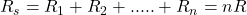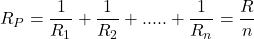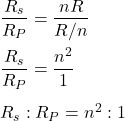## n resistance is each of resistance R is first connected in series and then in parallel what is the ratio of series and parallel combination​

Question

n resistance is each of resistance R is first connected in series and then in parallel what is the ratio of series and parallel combination​

in progress 0
5 months 2021-08-31T10:23:14+00:00 1 Answers 14 views 0

The ratio of the ratio of series and parallel combination​ is n²:1.

Explanation:

The equivalent resistance in case of series combination is given by :….(1)

The equivalent resistance in case of parallel combination is given by :….(2)

Dividing equation (1) and (2) we get :So, the ratio of the ratio of series and parallel combination​ is n²:1. Hence, this is the required solution.